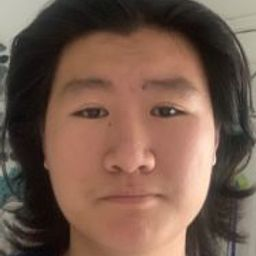Integrals

Vectors

Vector Functions

### Discussion

You must be signed in to discuss.
##### Top Calculus 3 Educators##### Heather Z.

Oregon State University##### Kristen K.

University of Michigan - Ann Arbor### Video Transcript

Okay, folks. So in this video, we have this line into grown. That we need to evaluate. This is this is problem number 11. We have this London grow and to grow along the curve C of X y plus why, plus Z Uh, yes. Okay. And were given x and y and see all of them as a function of t so excise the function of T is to t. Why, as a function of t is t and Z is to minus two t. Okay, So the way we're gonna do this is the usual way of evaluating line into girls which is by converting DS into something times DT. And if you remember and DS is just ex prime squared place. Why Prime Squared plus z prime squared The whole thing multiplied by DT and ex prime white Bar Mzee Prime, of course, are all just notation for a DX DT and D y d t indeed CDT. So what is the x DT? Well, the expertise to because X is to teach the dx tts you no two. So two squared plus the same thing with why but wise teas. And we have one squared plus minus two squared multiplied by D t. Now that's equal to four plus four plus one plus four, which is nice d t but nine. The square root of nine is just three. I hope you know that. So DS is three d t When a plant this back into the line integral. So we have the integral if along the curve C of x times What? What's x times? Y well x times Why is this thing times this thing? So that's those two t squared right to t squared. Plus what's why? What? Why is t and then added into Z? But he is two minus duty. Okay, that's nice. The S is three d t. I'm gonna pull the constant out in front. Now let's evaluate the line integral. So we have three ah to t squared minus two t plus ts minus t two plus two. Multiply it by DT. So now it's Let's evaluate this this integral here. So we have three of, um to over three t three minus one Hertie squared plus two t evaluated at the two other to our limits of integration. The 1st 1 to 0 and the top one is one. Let's do it. Let's plug in and let some do some plug and chug. Here we have three. I'm the, you know, plugging the one and zero, respectively. We have two of her, three minus 1/2 plus two. That's for the top limit minus 000 which is just zero. So this is gone. So we have, um let's multiply the three inside of the parentheses. So we have two minus three have plus two. Oh, excuse me. Not plus two plus two times three plus six. Okay, so So this is eight minus 3/2 which is, uh, a fine at 1.5, which is Ah, six point fire, which is 13 over to. This is the answer for this video. Thank you, but IUniversity of California, Berkeley

#### Topics

Integrals

Vectors

Vector Functions

##### Top Calculus 3 Educators##### Heather Z.

Oregon State University##### Kristen K.

University of Michigan - Ann Arbor